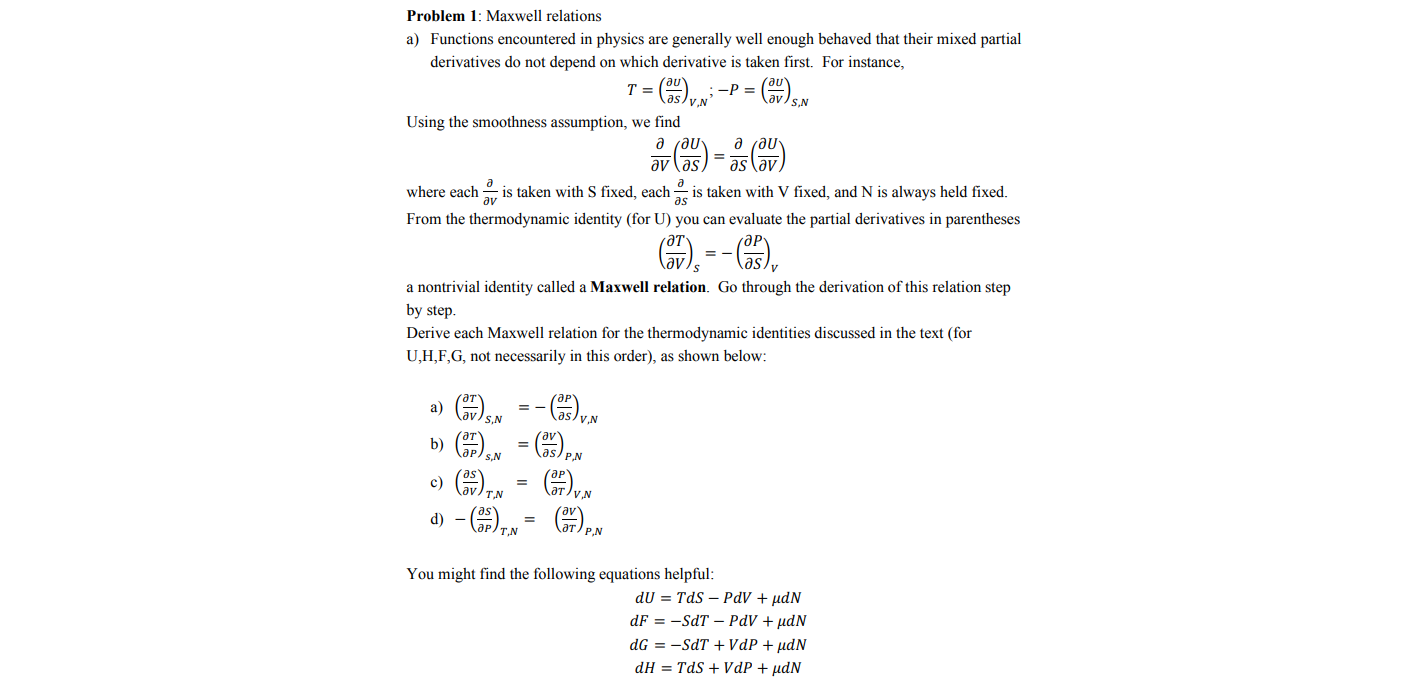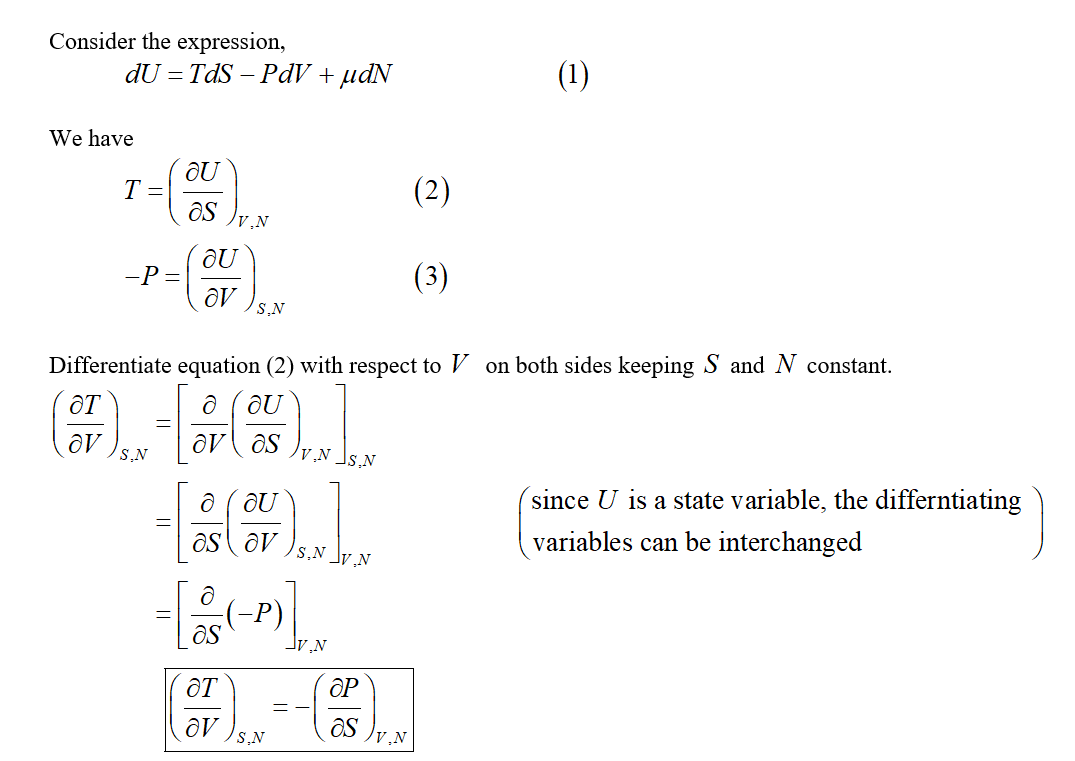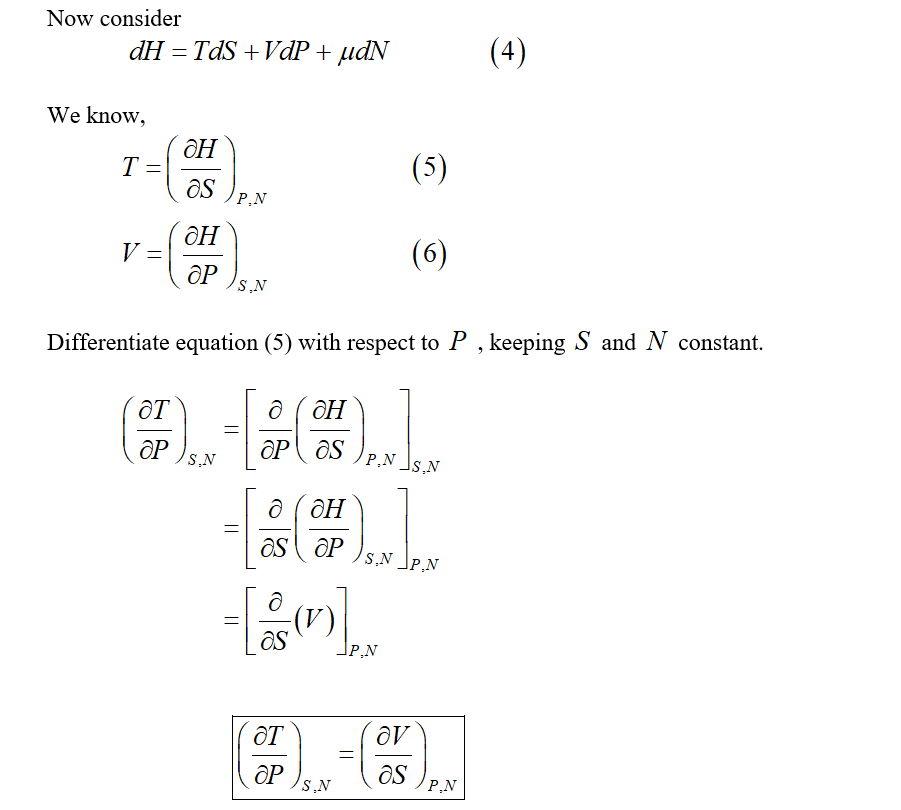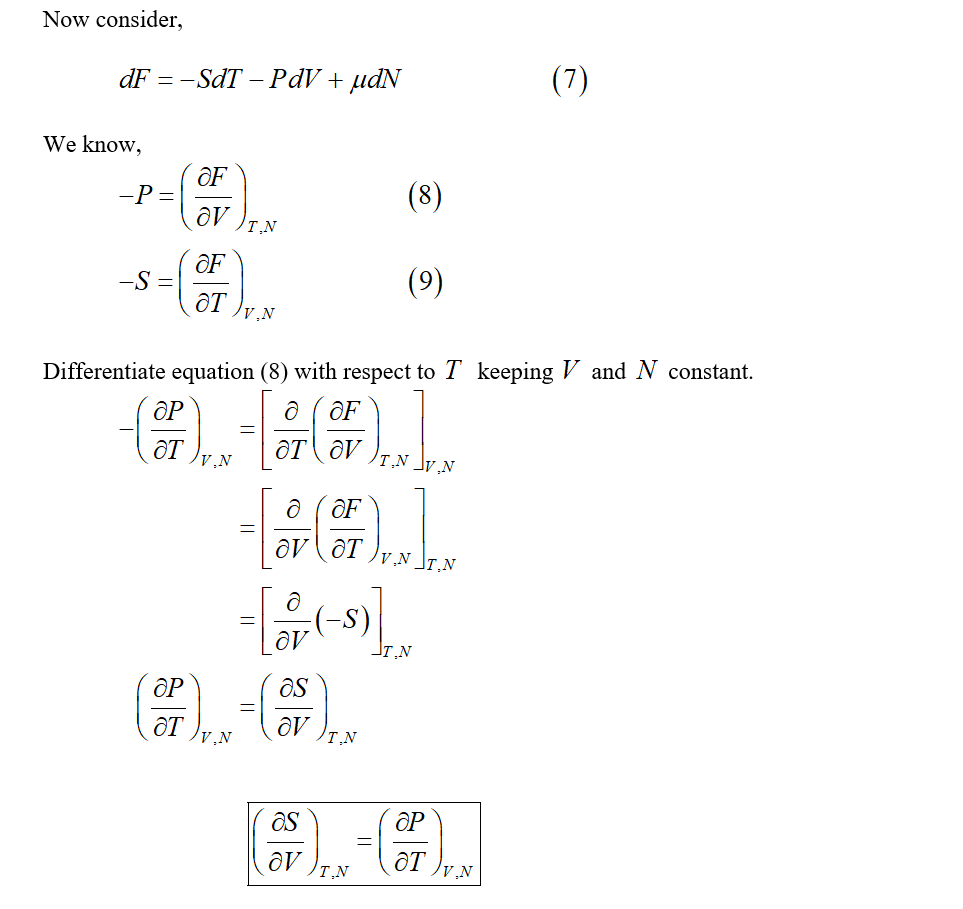# Problem 1: Maxwell relationsa) Functions encountered in physics are generally well enough behaved that their mixed partialderivatives do not depend on which derivative is taken first. For instance,-P =aslvNS,NUsing the smoothness assumption, we findд гди-where eachavis taken with S fixed, eachis taken with V fixed, and N is always held fixed.From the thermodynamic identity (for U) you can evaluate the partial derivatives in parenthesesa nontrivial identity called a Maxwell relation. Go through the derivation of this relation stepby step.Derive each Maxwell relation for the thermodynamic identities discussed in the text (forU,H,F,G, not necessarily in this order), as shown below:a)lavS,Nas/y.Nb)s/ P.NN*()d) - (),c)ат/уavат/ р NYou might find the following equations helpful:dU = TdS – PdV + µdNdF = -SdT – PdV + µdNdG = -SdT + VdP + µdNdH = TdS + VdP + µdN

Question
1 viewshelp_outlineImage TranscriptioncloseProblem 1: Maxwell relations a) Functions encountered in physics are generally well enough behaved that their mixed partial derivatives do not depend on which derivative is taken first. For instance, -P = aslvN S,N Using the smoothness assumption, we find д гди- where each av is taken with S fixed, each is taken with V fixed, and N is always held fixed. From the thermodynamic identity (for U) you can evaluate the partial derivatives in parentheses a nontrivial identity called a Maxwell relation. Go through the derivation of this relation step by step. Derive each Maxwell relation for the thermodynamic identities discussed in the text (for U,H,F,G, not necessarily in this order), as shown below: a) lav S,N as/y.N b) s/ P.N N*() d) - (), c) ат/у av ат/ р N You might find the following equations helpful: dU = TdS – PdV + µdN dF = -SdT – PdV + µdN dG = -SdT + VdP + µdN dH = TdS + VdP + µdN fullscreen
check_circle

Step 1Step 2Step 3...

### Want to see the full answer?

See Solution

#### Want to see this answer and more?

Solutions are written by subject experts who are available 24/7. Questions are typically answered within 1 hour.*

See Solution
*Response times may vary by subject and question.
Tagged in